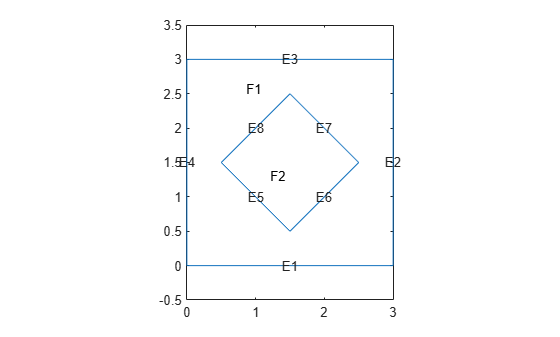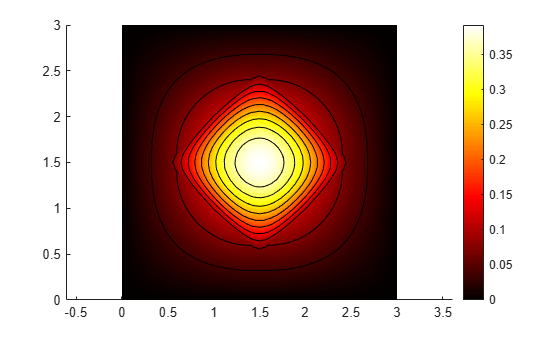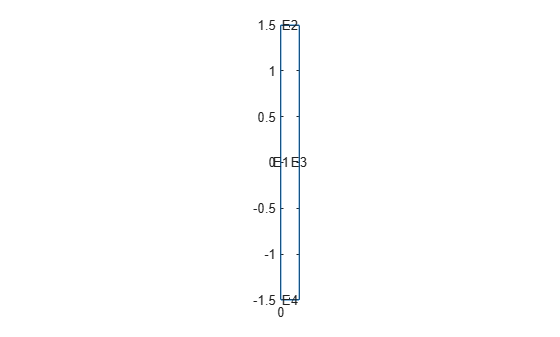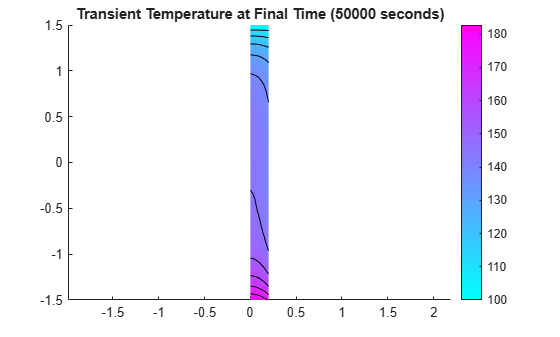# TransientThermalResults

Transient thermal solution and derived quantities

## Description

A `TransientThermalResults` object contains the temperature and gradient values in a form convenient for plotting and postprocessing.

The temperature and its gradient are calculated at the nodes of the triangular or tetrahedral mesh generated by `generateMesh`. Temperature values at the nodes appear in the `Temperature` property. The solution times appear in the `SolutionTimes` property. The three components of the temperature gradient at the nodes appear in the `XGradients`, `YGradients`, and `ZGradients` properties. You can extract solution and gradient values for specified time indices from `Temperature`, `XGradients`, `YGradients`, and `ZGradients`.

To interpolate the temperature or its gradient to a custom grid (for example, specified by `meshgrid`), use `interpolateTemperature` or `evaluateTemperatureGradient`.

To evaluate heat flux of a thermal solution at nodal or arbitrary spatial locations, use `evaluateHeatFlux`. To evaluate integrated heat flow rate normal to a specified boundary, use `evaluateHeatRate`.

## Creation

Solve a transient thermal problem using the `solve` function. This function returns a transient thermal solution as a `TransientThermalResults` object.

expand all

## All Transient Thermal Models

Finite element mesh, returned as an FEMesh Properties object.

Temperature values at nodes, returned as a vector or matrix.

Data Types: `double`

Solution times, returned as a real vector. `SolutionTimes` is the same as the `tlist` input to `solve`.

Data Types: `double`

## Non-Axisymmetric Models

x-component of the temperature gradient at nodes, returned as a vector or matrix.

Data Types: `double`

y-component of the temperature gradient at nodes, returned as a vector or matrix.

Data Types: `double`

z-component of the temperature gradient at nodes, returned as a vector or matrix.

Data Types: `double`

## Axisymmetric Models

r-component of the temperature gradient at nodes, returned as a vector or matrix.

Data Types: `double`

z-component of the temperature gradient at nodes, returned as a vector or matrix.

Data Types: `double`

## Object Functions

 `evaluateHeatFlux` Evaluate heat flux of a thermal solution at nodal or arbitrary spatial locations `evaluateHeatRate` Evaluate integrated heat flow rate normal to specified boundary `evaluateTemperatureGradient` Evaluate temperature gradient of a thermal solution at arbitrary spatial locations `interpolateTemperature` Interpolate temperature in a thermal result at arbitrary spatial locations

## Examples

collapse all

Solve a 2-D transient thermal problem.

Create a transient thermal model for this problem.

`thermalmodel = createpde('thermal','transient');`

Create the geometry and include it in the model.

```SQ1 = [3; 4; 0; 3; 3; 0; 0; 0; 3; 3]; D1 = [2; 4; 0.5; 1.5; 2.5; 1.5; 1.5; 0.5; 1.5; 2.5]; gd = [SQ1 D1]; sf = 'SQ1+D1'; ns = char('SQ1','D1'); ns = ns'; dl = decsg(gd,sf,ns); geometryFromEdges(thermalmodel,dl); pdegplot(thermalmodel,'EdgeLabels','on','FaceLabels','on') xlim([-1.5 4.5]) ylim([-0.5 3.5]) axis equal```For the square region, assign these thermal properties:

• Thermal conductivity is $10\text{\hspace{0.17em}}\mathrm{W}/\left(\mathrm{m}{\cdot }^{\circ }\mathrm{C}\right)$

• Mass density is $2\text{\hspace{0.17em}}\mathrm{kg}/{\mathrm{m}}^{3}$

• Specific heat is $0.1\text{\hspace{0.17em}}\mathrm{J}/\left({\mathrm{kg}\cdot }^{\circ }\mathrm{C}\right)$

```thermalProperties(thermalmodel,'ThermalConductivity',10, ... 'MassDensity',2, ... 'SpecificHeat',0.1, ... 'Face',1);```

For the diamond region, assign these thermal properties:

• Thermal conductivity is $2\text{\hspace{0.17em}}\mathrm{W}/\left(\mathrm{m}{\cdot }^{\circ }\mathrm{C}\right)$

• Mass density is $1\text{\hspace{0.17em}}\mathrm{kg}/{\mathrm{m}}^{3}$

• Specific heat is $0.1\text{\hspace{0.17em}}\mathrm{J}/\left({\mathrm{kg}\cdot }^{\circ }\mathrm{C}\right)$

```thermalProperties(thermalmodel,'ThermalConductivity',2, ... 'MassDensity',1, ... 'SpecificHeat',0.1, ... 'Face',2);```

Assume that the diamond-shaped region is a heat source with a density of $4\text{\hspace{0.17em}}\mathrm{W}/{\mathrm{m}}^{2}$.

`internalHeatSource(thermalmodel,4,'Face',2);`

Apply a constant temperature of $0{\phantom{\rule{0.16666666666666666em}{0ex}}}^{\circ }C$ to the sides of the square plate.

`thermalBC(thermalmodel,'Temperature',0,'Edge',[1 2 7 8]);`

Set the initial temperature to 0 °C.

`thermalIC(thermalmodel,0);`

Generate the mesh.

`generateMesh(thermalmodel);`

The dynamics for this problem are very fast. The temperature reaches a steady state in about 0.1 seconds. To capture the interesting part of the dynamics, set the solution time to `logspace(-2,-1,10)`. This command returns 10 logarithmically spaced solution times between 0.01 and 0.1.

`tlist = logspace(-2,-1,10);`

Solve the equation.

`thermalresults = solve(thermalmodel,tlist)`
```thermalresults = TransientThermalResults with properties: Temperature: [1481x10 double] SolutionTimes: [1x10 double] XGradients: [1481x10 double] YGradients: [1481x10 double] ZGradients: [] Mesh: [1x1 FEMesh] ```

Plot the solution with isothermal lines by using a contour plot.

```T = thermalresults.Temperature; pdeplot(thermalmodel,'XYData',T(:,10),'Contour','on','ColorMap','hot')```Analyze heat transfer in a rod with a circular cross-section and internal heat generation by simplifying a 3-D axisymmetric model to a 2-D model.

Create a transient thermal model for solving an axisymmetric problem.

`thermalmodel = createpde('thermal','transient-axisymmetric');`

The 2-D model is a rectangular strip whose x-dimension extends from the axis of symmetry to the outer surface and whose y-dimension extends over the actual length of the rod (from `-`1.5 m to 1.5 m). Create the geometry by specifying the coordinates of its four corners. For axisymmetric models, the toolbox assumes that the axis of rotation is the vertical axis passing through r = 0.

`g = decsg([3 4 0 0 .2 .2 -1.5 1.5 1.5 -1.5]');`

Include the geometry in the model.

`geometryFromEdges(thermalmodel,g);`

Plot the geometry with the edge labels.

```figure pdegplot(thermalmodel,'EdgeLabels','on') axis equal```The rod is composed of a material with these thermal properties.

```k = 40; % thermal conductivity, W/(m*C) rho = 7800; % density, kg/m^3 cp = 500; % specific heat, W*s/(kg*C) q = 20000; % heat source, W/m^3```

Specify the thermal conductivity, mass density, and specific heat of the material.

```thermalProperties(thermalmodel,'ThermalConductivity',k,... 'MassDensity',rho,... 'SpecificHeat',cp);```

Specify internal heat source and boundary conditions.

`internalHeatSource(thermalmodel,q);`

Define the boundary conditions. There is no heat transferred in the direction normal to the axis of symmetry (edge 1). You do not need to change the default boundary condition for this edge. Edge 2 is kept at a constant temperature T = 100 °C.

`thermalBC(thermalmodel,'Edge',2,'Temperature',100);`

Specify the convection boundary condition on the outer boundary (edge 3). The surrounding temperature at the outer boundary is 100 °C, and the heat transfer coefficient is $50\text{\hspace{0.17em}}\mathrm{W}/\left(\mathrm{m}{\cdot }^{\circ }\mathrm{C}\right)$.

```thermalBC(thermalmodel,'Edge',3,... 'ConvectionCoefficient',50,... 'AmbientTemperature',100);```

The heat flux at the bottom of the rod (edge 4) is $5000\text{\hspace{0.17em}}\mathrm{W}/{\mathrm{m}}^{2}$.

`thermalBC(thermalmodel,'Edge',4,'HeatFlux',5000);`

Specify that the Initial temperature in the rod is zero.

`thermalIC(thermalmodel,0);`

Generate the mesh.

`generateMesh(thermalmodel);`

Compute the transient solution for solution times from `t = 0` to `t = 50000` seconds.

```tfinal = 50000; tlist = 0:100:tfinal; result = solve(thermalmodel,tlist)```
```result = TransientThermalResults with properties: Temperature: [259x501 double] SolutionTimes: [1x501 double] RGradients: [259x501 double] ZGradients: [259x501 double] Mesh: [1x1 FEMesh] ```

Plot the temperature distribution at `t = 50000` seconds.

```T = result.Temperature; figure pdeplot(thermalmodel,'XYData',T(:,end),'Contour','on') axis equal title(sprintf('Transient Temperature at Final Time (%g seconds)',tfinal))```Introduced in R2017a

## Support평가판 신청